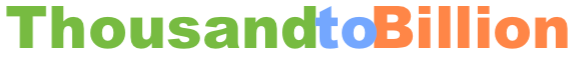Convert 50 thousand to billionHere we will convert 50 thousand to billion. In other words, what is 50 thousand in terms of billion? We start by showing you how to write 50 thousand with numbers:

50,000

There are one million thousand in a billion. To give you perspective, below is how to write a billion in numbers.

1,000,000,000

And here is how our 50 thousand fits into a billion, which will illustrate how much larger a billion is compared to 50 thousand.

1,000,050,000

Since there are one million thousand in a billion, to convert 50 thousand to billion, you would divide 50 thousand by one million. Here is the math and the answer:

50 / 1,000,000 = 0.00005
50 thousand = 0.00005 billion

Thousand to Billion Converter
We can do more! Convert another amount of thousand to billion here.

Convert 50.1 thousand to billion
Here is the next amount of thousand on our list that we have converted to billion.